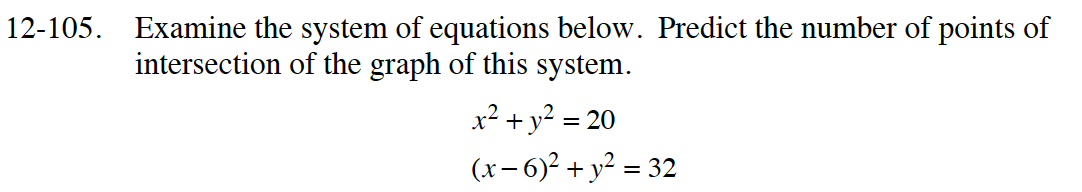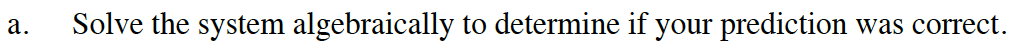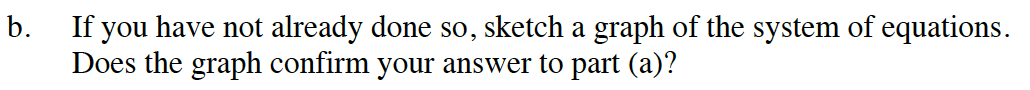### Home > INT2 > Chapter 12 > Lesson 12.2.3 > Problem12-105

12-105.
1. Examine the system of equations below. Predict the number of points of intersection of the graph of this system. Homework Help ✎

x2 + y2 = 20
(x − 6)2 + y2 = 32

1. Solve the system algebraically to determine if your prediction was correct.

2. If you have not already done so, sketch a graph of the system of equations. Does the graph confirm your answer to part (a)?To solve algebraically, solve the top equation for y2 and then substitute.The circles should intersect twice.